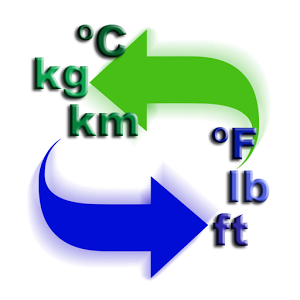# Atomic Units Converters

## Atomic Energy Converter

 Hartrees: eV: kJ/mol: kcal/mol: cm-1: K:

### Relationships Used :

 1 Hartree (Eh) = 27.21138602 eV = 4.359744650×10−18 J = 627.509474 kcal/mol = 2625.499638 kJ/mol = 219474.6313702 cm−1 = 6579.683920711 THz = 315775.13 K

## Atomic Length Converter

 Bohrs = a.u. Å (Ångströms) pm (Picometers) nm (Nanometers) μ; μm (Micrometers)

Relationship used: 1 Bohr = 5.2917721067×10−11 m 

## Temperature Converter

 K (Kelvins): °C (Celsius): °F (Fahrenheits): °R (Rankines):

 K = °C + 273.15 = (°F + 459.67) * (5/9) = °R * (5/9) °C = K - 273.15 = (°F-32)(5/9) = (°R - 491.67) * (5/9) °F = K * (9/5) - 459.67 = (9/5)°C + 32 = °R - 459.67 °R = K * (9/5) = (°C + 273.15) * (9/5) = °F + 459.67

Disclaimer: The information contained in this website is provided in the hope that it will be useful, but WITHOUT ANY WARRANTY; without even the implied warranty of MERCHANTABILITY or FITNESS FOR A PARTICULAR PURPOSE. See the GNU General Public License for more details. By using our website, you hereby consent to our disclaimer and agree to its terms.